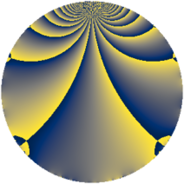# Properties

 Label 1143.2.aLevel $1143$ Weight $2$ Character orbit 1143.a Rep. character $\chi_{1143}(1,\cdot)$ Character field $\Q$ Dimension $53$ Newform subspaces $11$ Sturm bound $256$ Trace bound $7$

# Related objects

## Defining parameters

 Level: $$N$$ $$=$$ $$1143 = 3^{2} \cdot 127$$ Weight: $$k$$ $$=$$ $$2$$ Character orbit: $$[\chi]$$ $$=$$ 1143.a (trivial) Character field: $$\Q$$ Newform subspaces: $$11$$ Sturm bound: $$256$$ Trace bound: $$7$$ Distinguishing $$T_p$$: $$2$$, $$5$$, $$7$$

## Dimensions

The following table gives the dimensions of various subspaces of $$M_{2}(\Gamma_0(1143))$$.

Total New Old
Modular forms 132 53 79
Cusp forms 125 53 72
Eisenstein series 7 0 7

The following table gives the dimensions of the cuspidal new subspaces with specified eigenvalues for the Atkin-Lehner operators and the Fricke involution.

$$3$$$$127$$FrickeDim.
$$+$$$$+$$$$+$$$$6$$
$$+$$$$-$$$$-$$$$16$$
$$-$$$$+$$$$-$$$$18$$
$$-$$$$-$$$$+$$$$13$$
Plus space$$+$$$$19$$
Minus space$$-$$$$34$$

## Trace form

 $$53q + 50q^{4} + 4q^{5} + 2q^{7} + 6q^{8} + O(q^{10})$$ $$53q + 50q^{4} + 4q^{5} + 2q^{7} + 6q^{8} + 4q^{10} - 8q^{11} + 6q^{13} + 44q^{16} + 4q^{17} + 2q^{19} + 12q^{20} + 2q^{22} + 10q^{23} + 63q^{25} + 16q^{26} + 12q^{28} + 6q^{29} + 4q^{31} + 27q^{32} + 5q^{34} + 4q^{35} + 4q^{37} - q^{38} + 8q^{40} + 10q^{43} - 19q^{44} - 26q^{46} - 22q^{47} + 63q^{49} + 8q^{50} + 7q^{52} - 6q^{53} + 6q^{55} - 16q^{56} - 18q^{58} - 4q^{59} + 6q^{61} + 45q^{62} + 2q^{64} + 22q^{65} + 16q^{67} - 6q^{68} - 22q^{70} - 46q^{71} + 22q^{73} + 21q^{74} + 4q^{76} + 12q^{77} - 6q^{79} + 88q^{80} - 4q^{82} - 18q^{83} - 6q^{85} - 24q^{86} - 33q^{88} + 18q^{89} - 20q^{91} + 62q^{92} - 66q^{94} + 48q^{95} + 66q^{97} + 38q^{98} + O(q^{100})$$

## Decomposition of $$S_{2}^{\mathrm{new}}(\Gamma_0(1143))$$ into newform subspaces

Label Dim. $$A$$ Field CM Traces A-L signs $q$-expansion
$$a_2$$ $$a_3$$ $$a_5$$ $$a_7$$ 3 127
1143.2.a.a $$1$$ $$9.127$$ $$\Q$$ None $$-2$$ $$0$$ $$-3$$ $$-4$$ $$-$$ $$+$$ $$q-2q^{2}+2q^{4}-3q^{5}-4q^{7}+6q^{10}+\cdots$$
1143.2.a.b $$1$$ $$9.127$$ $$\Q$$ None $$0$$ $$0$$ $$-1$$ $$0$$ $$+$$ $$+$$ $$q-2q^{4}-q^{5}+4q^{11}+q^{13}+4q^{16}+\cdots$$
1143.2.a.c $$1$$ $$9.127$$ $$\Q$$ None $$0$$ $$0$$ $$1$$ $$-2$$ $$-$$ $$-$$ $$q-2q^{4}+q^{5}-2q^{7}+4q^{11}-3q^{13}+\cdots$$
1143.2.a.d $$1$$ $$9.127$$ $$\Q$$ None $$0$$ $$0$$ $$1$$ $$0$$ $$+$$ $$+$$ $$q-2q^{4}+q^{5}-4q^{11}+q^{13}+4q^{16}+\cdots$$
1143.2.a.e $$3$$ $$9.127$$ $$\Q(\zeta_{18})^+$$ None $$3$$ $$0$$ $$6$$ $$-3$$ $$-$$ $$+$$ $$q+(1-\beta _{1})q^{2}+(1-2\beta _{1}+\beta _{2})q^{4}+(2+\cdots)q^{5}+\cdots$$
1143.2.a.f $$4$$ $$9.127$$ $$\Q(\sqrt{3}, \sqrt{7})$$ None $$0$$ $$0$$ $$0$$ $$-6$$ $$+$$ $$+$$ $$q+(\beta _{1}+\beta _{3})q^{2}+q^{4}-\beta _{1}q^{5}+(-1+\cdots)q^{7}+\cdots$$
1143.2.a.g $$5$$ $$9.127$$ 5.5.246832.1 None $$-2$$ $$0$$ $$-1$$ $$0$$ $$-$$ $$-$$ $$q-\beta _{2}q^{2}+(\beta _{1}+\beta _{2}+\beta _{3}+\beta _{4})q^{4}+\cdots$$
1143.2.a.h $$5$$ $$9.127$$ 5.5.81509.1 None $$1$$ $$0$$ $$5$$ $$0$$ $$-$$ $$+$$ $$q+\beta _{1}q^{2}+\beta _{2}q^{4}+(1-\beta _{1}+\beta _{2}+\beta _{4})q^{5}+\cdots$$
1143.2.a.i $$7$$ $$9.127$$ $$\mathbb{Q}[x]/(x^{7} - \cdots)$$ None $$-2$$ $$0$$ $$-8$$ $$-3$$ $$-$$ $$-$$ $$q-\beta _{1}q^{2}+(1+\beta _{2})q^{4}+(-1+\beta _{4})q^{5}+\cdots$$
1143.2.a.j $$9$$ $$9.127$$ $$\mathbb{Q}[x]/(x^{9} - \cdots)$$ None $$2$$ $$0$$ $$4$$ $$10$$ $$-$$ $$+$$ $$q+\beta _{1}q^{2}+(2+\beta _{2})q^{4}+\beta _{5}q^{5}+(1-\beta _{8})q^{7}+\cdots$$
1143.2.a.k $$16$$ $$9.127$$ $$\mathbb{Q}[x]/(x^{16} - \cdots)$$ None $$0$$ $$0$$ $$0$$ $$10$$ $$+$$ $$-$$ $$q+\beta _{1}q^{2}+(1+\beta _{2})q^{4}-\beta _{14}q^{5}+(1+\cdots)q^{7}+\cdots$$

## Decomposition of $$S_{2}^{\mathrm{old}}(\Gamma_0(1143))$$ into lower level spaces

$$S_{2}^{\mathrm{old}}(\Gamma_0(1143)) \cong$$ $$S_{2}^{\mathrm{new}}(\Gamma_0(127))$$$$^{\oplus 3}$$$$\oplus$$$$S_{2}^{\mathrm{new}}(\Gamma_0(381))$$$$^{\oplus 2}$$